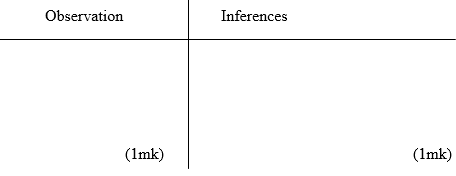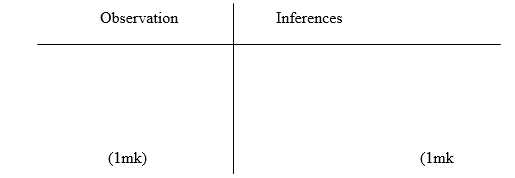##### Search### KCSE CLUSTER TEST 14

#### Chemistry Paper 3

1.

You are provided with:

- 4.5g of solid K in a boiling tube.

- Solution L, 0.06M acidified Potassium manganate (VII).

You are required to determine

1) The solubility of solid K at different temperatures.

2) The number of moles of water of crystallization in solid K. Procedure a) Using a burette, add 4cm3 of distilled water to solid K in the boiling tube.

Heat the mixture while stirring with the thermometer to about 700C.

When all the solid has dissolved, allow the solution to cool while stirring with the thermometer. Note the temperature at which crystals of solid K first appear.

b) Using the burette, add 2cm3 of distilled water to the contents of the boiling tube.

Warm the mixture while stirring with the thermometer until all the solid dissolves. Allow the mixture to cool while stirring. Note and record the temperature at which crystals of solid K first appear.

c) Repeat procedure (b) two more times and record the temperature in table

1. Retain the contents of the boiling tube for use in procedure (e)

d) i) Complete table I by calculating the solubility of solid K at the different temperatures.

The solubility of a substance is the mass of that substance that dissolves in 100cm3 (100g) of water at a particular temperature.

Table Iii) On the grid provided, plot a graph of solubility of solid K (vertical axis) against temperature.iii) Using your graph, determine the temperature, 100g of solid K would dissolve in 100cm3 of water. (1mk)

e) i) Transfer the contents of the boiling tube into a 250ml volumetric flask.

Rinse both the boiling tube and the thermometer with distilled water and add to the volumetric flask

. Add more distilled water to make up to the mark.

Label this solution K.

Fill the burette with solution L.

Using a pipette and pipette filler, place 25.0cm3 of solution K into a conical flask.

Warm the mixture to about 600C.

Titrate the hot solution K with solution L until a permanent pink colour appears.

Record your readings in table 2. Repeat the titration two more times and complete table 2

ii) Calculate the I. average volume of solution L used

II. number of moles of potassium manganate (VII) used

III. number of moles of K in 25.0cm3 of solution K given that 2 moles of potassium manganate (VII) react completely with 5 moles of K.

IV. relative formula mass of K

iii) The formula of K has the form G.nH2O. Determine the value of n in the formula
given that the relative formula mass of G is 90.0 (O =16, H=1.0).

21 marks

2.

You are provided with solid P

You are required to: ACIDS BASES AND SALTS

i) Carry out the tests described below. ii) Write your observations and inferences accordingly.

Procedure

- Put solid P provided in a clean boiling tube, add about 10cm3 of distilled water and shake well.

- Divide the resulting solution into five portions

i) Add 5 drops of 2M sodium hydroxide solution to the first portionii) Dip a clean glass rod in the second portion, then place it on a Bunsen burner flameiii) Add 3 drops of lead (II) nitrate solution to the third portion.iv) Add about 2cm3 of Barium (II) Chloride solution, to the 4th portion, followed by about 5cm3 of 2M hydrochloric acid.v) Add 5 drops of acidified potassium dichromate (VI) to the fifth portion.11 marks

3.

You are provided with solid Q. Carry out the tests in (a) and (b) and write your observations and inferences in the spaces provided.

I. Place about one third of solid Q on a metallic spatula and burn it in a Bunsen burner flame.II. Dissolve all the remaining solid Q in about 10cm3 of distilled water in a boiling tube. Use the solution for tests (b) (i), (ii) and (iii). i) Place 2cm3 of the solution in a test – tube and add 2 drops of acidified potassium manganate (VII)ii) To 2cm3 of the solution, add all of solid sodium hydrogen carbonate provided.iii) To 2cm3 of the solution, add 2 drops of Bromine water.8 marks

Back Top